# RRphylo

## RRphylo Basics

RRphylo is a full-fledged phylogenetic comparative method, based on RidgeRace regression (Kratsch and McHardy, 2014). It provides the rate of phenotypic change per branch and the vector of phenotypic estimates at nodes, with either continuous or discrete, univariate or multivariate data (Castiglione et al. 2018, 2020a). As compared to classic, model-based approaches, RRphylo makes no a priori assumption about the tempo and mode of phenotypic evolution. However, rates are presumed to change more between than within clades (see below) and the rate of change is calculated as proportional to time (branch lengths).

### RRphylo phylogenetic ridge regression

The RRphylo method is based on RidgeRace regression from Kratsch and McHardy (2014). Under both methods, the phenotypic change between a node and a daughter tip along a phyletic line is described by the sum of individual contributions at each consecutive branch according to the equation: $Δy = β_{1}*l_{1} + β_{2}*l_{2} + ... β_{n}*l_{n}$ where $$n$$ equals the number of branches intervening between the node and the tip, $$β_{1...n}$$ are the vectors of regression coefficients (the evolutionary rates) at each branch, and $$l_{1...n}$$ are the branch lengths. Extending this calculation simultaneously for the entire phylogeny, the $$\hat{β}$$ vector is calculated as:

betas <- (solve(t(L) %*% L + lambda * diag(ncol(L))) %*% t(L)) %*% as.matrix(y)

and the vector of predicted phenotypes at tips and nodes, respectively:

y.pred <- L %*% betas
ace <- L1 %*% betas[1:Nnode(tree),]

Where L and L1 are matrices of root to tip distances and λ is a penalization factor.

L is a $$n*m$$ matrix, where n = number of tips and m = number of branches (i.e. number of tips + number of nodes). Each row represents the branch lengths aligned along a root-to-tip path.

L1 is a $$m*m$$ matrix, where m = number of internal branches (i.e. number of nodes). Each row represents the branch lengths aligned along a root-to-node path.

require(ape)
set.seed(76)
rtree(5)->tree
makeL(tree)->L
makeL1(tree)->L1

plot(tree,no.margin=TRUE,edge.width=1.7)
nodelabels(bg="w",frame="n",col="red",adj=-0.01)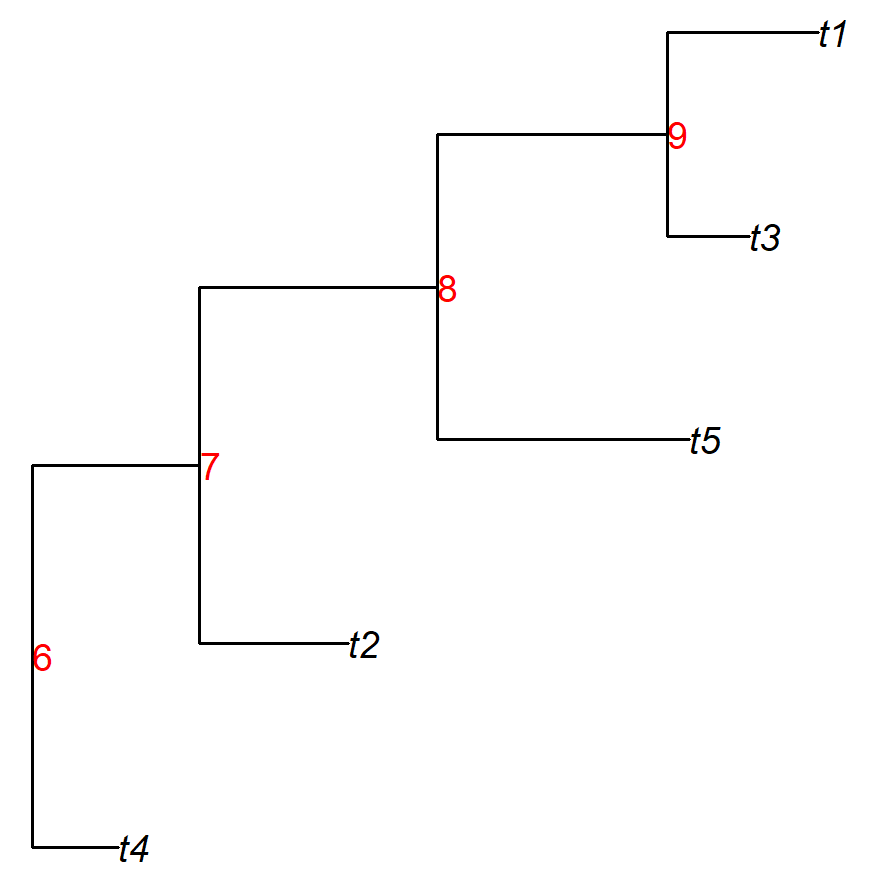L1 matrix
6 7 8 9
6 1 0.000 0.000 0.000
7 1 0.627 0.000 0.000
8 1 0.627 0.888 0.000
9 1 0.627 0.888 0.861
L matrix
6 7 8 9 t4 t2 t5 t3 t1
t4 1 0.000 0.000 0.000 0.322 0.000 0.000 0.000 0.000
t2 1 0.627 0.000 0.000 0.000 0.557 0.000 0.000 0.000
t5 1 0.627 0.888 0.000 0.000 0.000 0.943 0.000 0.000
t3 1 0.627 0.888 0.861 0.000 0.000 0.000 0.309 0.000
t1 1 0.627 0.888 0.861 0.000 0.000 0.000 0.000 0.568

The λ value penalizes the fit of predicted phenotypes by adding a penalty on large values of $$β$$ as to avoid overparameterization and multicollinearity. In fact, when λ is close to 0, the $$\hat{β}$$ vector is calculated as to compute the phenotypic vector perfectly:

require(phytools)

rtree(100)->tree
makeL(tree)->L

# produce a phenotypic vector for both tips and nodes
fastBM(tree,internal=T)->phen
phen[1:100]->y
phen[101:199]->acey

# set λ close to 0
lambda <-1e-10

# compute evolutionary rates and estimate phenotypic values at tips as within RRphylo
betas<-(solve(t(L) %*% L +  lambda *diag(ncol(L))) %*% t(L)) %*% as.matrix(y)
y.pred <- L %*% betas

plot(y,y.pred,pch=21,bg="gray80",cex=1.5,mgp=c(1.8,0.5,0),
xlab="simulated",ylab="estimated",main="phenotype at tips")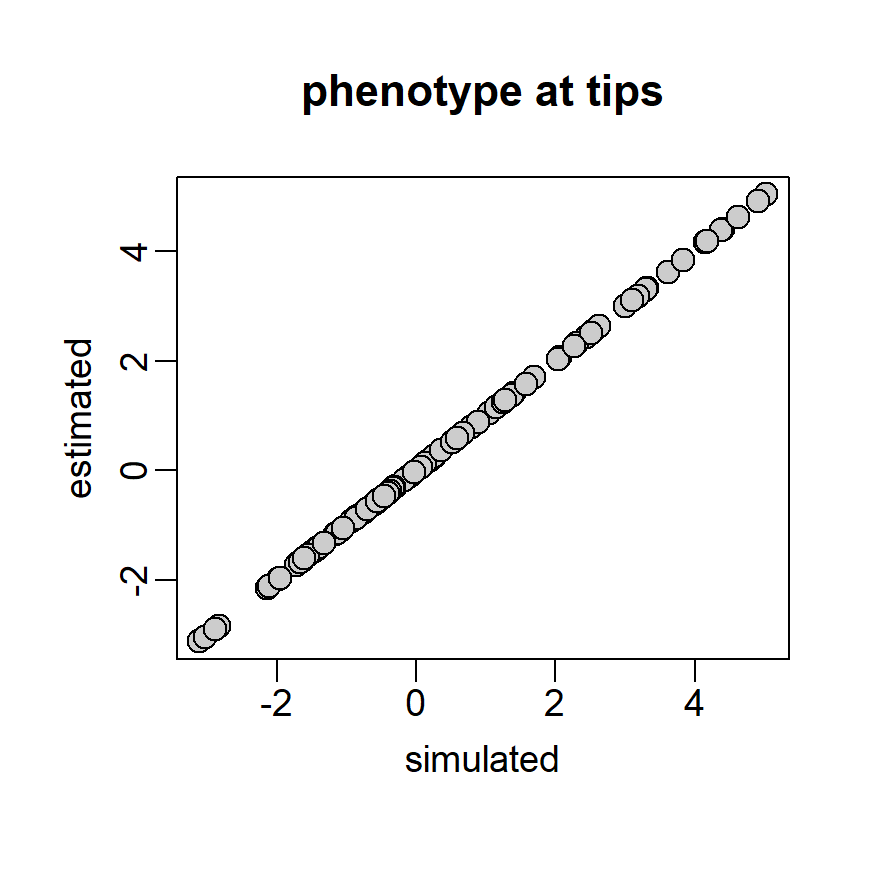Yet, such perfect fitting corresponds to a phylogeny with many phenotypic rates at zero and only few branches with rates of high absolute values, describing a rather implausible model for phenotypic evolution. Also, it makes it impossible to estimate the ancestral character values (aces) at internal nodes.

par(mfrow=c(1,2),mar=c(4,3,3,1))

plot(c(acey,y),betas,bg=c(rep("red",99),rep("green",100)),pch=21,cex=1.5,mgp=c(1.8,0.5,0),
xlab="phenotypes at nodes and tips",ylab="rates",main="rates vs simulated phenotypes")
legend("topright",legend=c("nodes","tips"),fill=c("red","green"),bty="n")

# compute ancestral character estimates at nodes as within RRphylo
makeL1(tree)->L1
ace <- L1 %*% betas[1:Nnode(tree),]
plot(acey,ace,pch=21,bg="gray80",cex=1.5,mgp=c(1.8,0.5,0),
xlab="simulated",ylab="estimated",main="phenotype at nodes (aces)")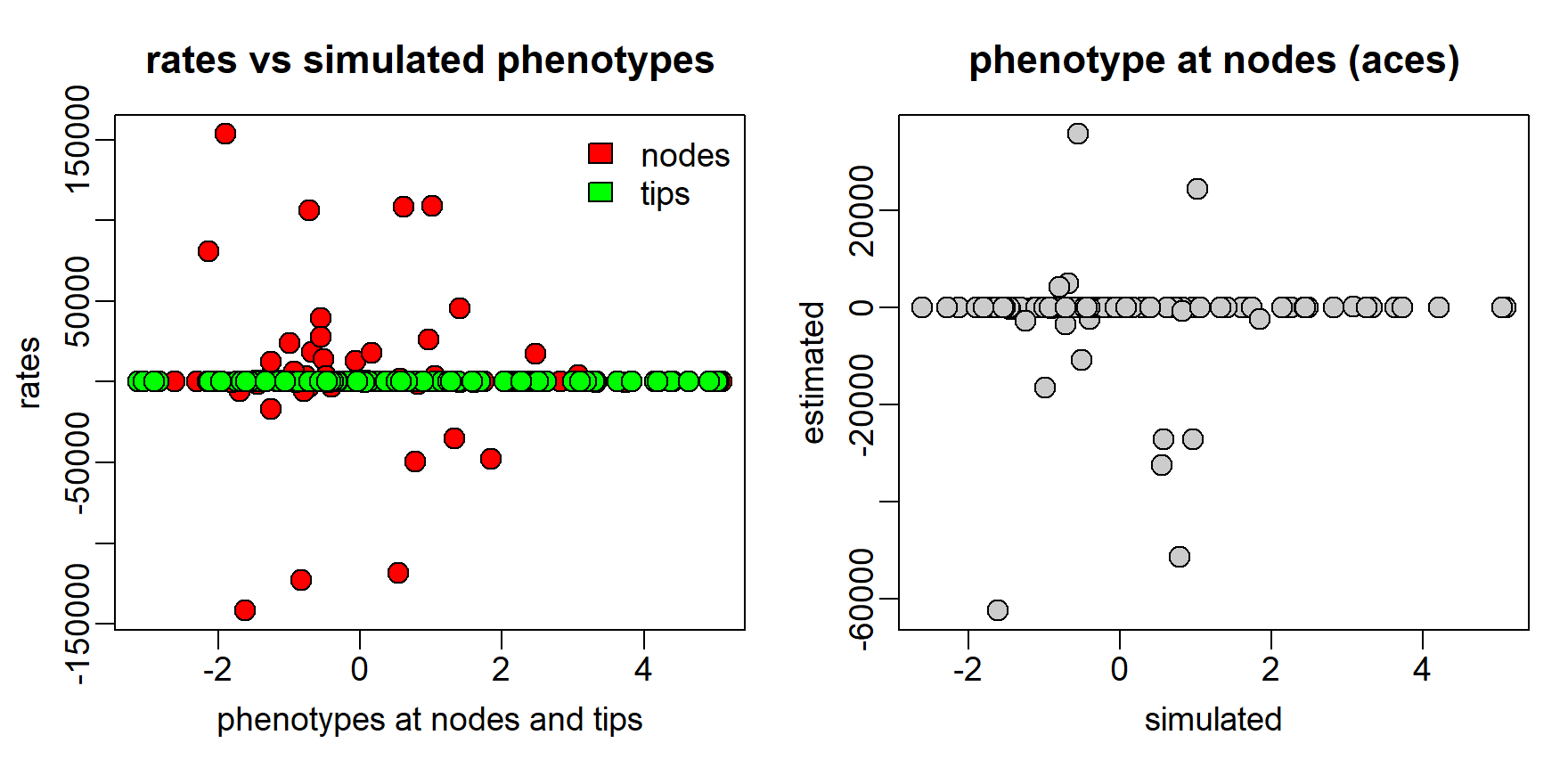In Kratsch and McHardy (2014), λ is estimated through L2 (quadratic) penalization. Within RRphylo (Castiglione et al. 2018), λ is fitted by an inner function optL, which is written as to minimize the rate variation within clades, thereby acting conservatively in terms of the chance to find rate shifts and introducing phylogenetic autocorrelation in evolutionary rates (Sakamoto & Venditti, Eastmann et al. 2011) .

RRphylo(tree,y)->RR
RR$rates[,1]->betas RR$predicted.phenotype[,1]->y.pred
c(RR$aces[,1],y)->cov # within RRphylo the logged absolute rates are regressed against # the absolute covariate values, and regression residuals are used # as rate values R <- log(abs(betas)) Y <- abs(cov) res <- residuals(lm(R ~ Y)) betas <- as.matrix(res) par(mfrow=c(1,2),mar=c(4,3,3,1)) plot(Y,R,pch=21,bg="gray80",cex=1.5,mgp=c(1.8,0.5,0), xlab="absolute covariate values",ylab="log absolute rates") abline(lm(R~Y),lwd=2,col="red") plot(Y,res,pch=21,bg="gray80",cex=1.5,mgp=c(1.8,0.5,0), xlab="absolute covariate values",ylab="regression residuals") abline(lm(res~Y),lwd=2,col="red")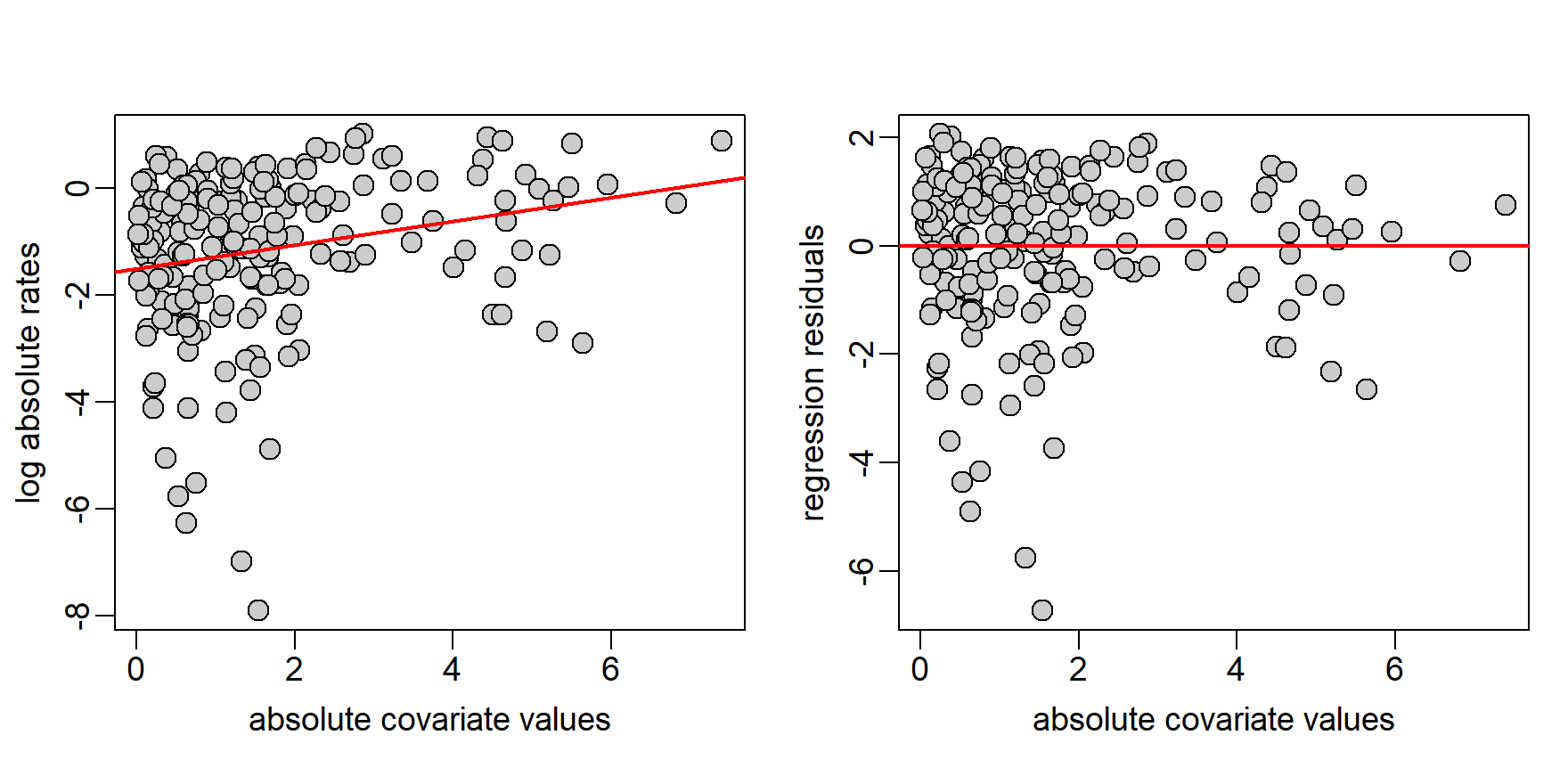## Multiple regression framework The multiple regression version of RRphylo is meant to evaluate the combined effect of phylogeny (basic predictor within RRphylo) and one to multiple additional predictors, either continuous or discrete (Castiglione et al. 2020a), on the evolution of a given phenotypic trait. Such additional predictor/s is/are included into the phylogenetic ridge regression equation as supplemental column/s within the L and L1 matrices. To associate predictor values to the L1 matrix (i.e. matrix of root-to-node paths), predictor values at nodes must be reconstructed. This is accomplished by performing RRphylo on the individual predictor/s, deriving their phenotypic estimates at nodes, and collating them to the values at tips. The final vector/matrix is fed into the RRphylo as the x1 argument.L1’ matrix 6 7 8 9 pred 6 1 0.000 0.000 0.000 -0.300 7 1 0.627 0.000 0.000 -0.488 8 1 0.627 0.888 0.000 -1.046 9 1 0.627 0.888 0.861 -1.690 L’ matrix 6 7 8 9 t4 t2 t5 t3 t1 pred t4 1 0.000 0.000 0.000 0.322 0.000 0.000 0.000 0.000 -0.255 t2 1 0.627 0.000 0.000 0.000 0.557 0.000 0.000 0.000 -0.417 t5 1 0.627 0.888 0.000 0.000 0.000 0.943 0.000 0.000 -0.902 t3 1 0.627 0.888 0.861 0.000 0.000 0.000 0.309 0.000 -1.629 t1 1 0.627 0.888 0.861 0.000 0.000 0.000 0.000 0.568 -2.174 This way, the vector $$\hat{β}$$ of rates is calculated for all the branches in the tree and the last element of $$\hat{β}$$ represents the partial phylogenetic ridge regression coefficient of the additional predictor, whereas the estimation of ancestral states remains unaffected. The multiple regression version of RRphylo is useful when a trait or ecological factor (i.e. the predictor) is presumed to influence the response variable and hence its evolutionary rates. One example is the effect of body size on brain size (Serio et al. 2019, Melchionna et al. 2020, see example below). ## Setting values at internal nodes There is widespread acknowledgement that the inclusion of fossil information in analyses of phenotypic and taxonomic diversification increases both the power and reliability of inference about the tempo and mode of evolution. RRphylo allows to integrate the phenotypic information at internal nodes in the estimation of evolutionary rates and ancestral character states (Castiglione et al. 2020b). Given a vector (or matrix in case of multivariate data) of $$n$$ known phenotypic values to be placed at internal nodes (aces argument within the function), a vector of false tips $$ftips$$ of length $$n$$ is added to the tree. Each $$i_{th}$$ element of $$ftips$$ is phenotypically identical to the corresponding $$aces_{i}$$ and is attached to the tree at the position of $$aces_{i}$$ with a branch of length = 0. Then, the vector $$\hat{β}$$ of regression coefficients is estimated by means of RRphylo by using the modified tree and phenotype (which include both $$ftips$$ and the real tips). Since the branch lengths of $$ftips$$ are equal to zero, the phenotypic rate between each $$ftips_{i}$$ and the corresponding node is zero, which means the $$aces$$ and their corresponding $$ftips$$ will have the same phenotypic estimates. After $$β$$ coefficients are estimated, the vector of phenotypic values at nodes is calculated as usual as L1 %*% betas[1:Nnode(tree),]. The final step of the algorithm consists in removing $$ftips$$ from the tree, and from the rate and phenotypic vectors.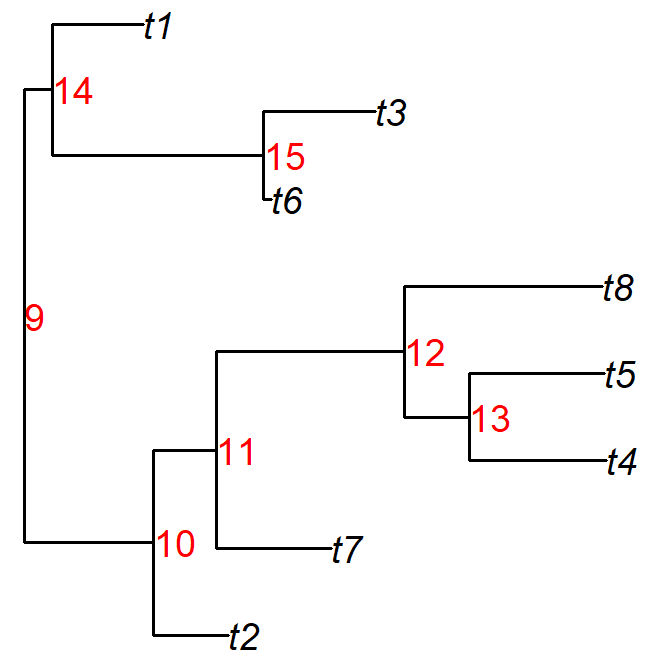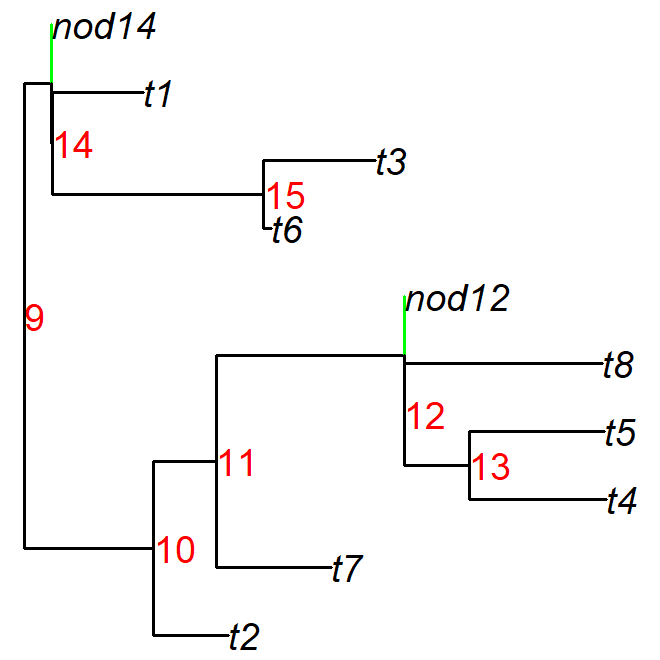## Guided examples # load the RRphylo example dataset including Cetaceans tree and data data("DataCetaceans") DataCetaceans$treecet->treecet # phylogenetic tree
DataCetaceans$masscet->masscet # logged body mass data DataCetaceans$brainmasscet->brainmasscet # logged brain mass data
DataCetaceans$aceMyst->aceMyst # known phenotypic value for the most recent # common ancestor of Mysticeti require(geiger) par(mar=c(0,0,0,1)) plot(ladderize(treecet,FALSE),show.tip.label = FALSE,edge.color = "gray40",edge.width = 1.5) plotinfo<-get("last_plot.phylo",envir =ape::.PlotPhyloEnv) nodelabels(text="",node=128,frame="circle",bg="red",cex=0.5) nodelabels(text="Mystacodon",node=128,frame="n",bg="w",cex=1,adj=c(-0.1,0.5),font=2) range(plotinfo$yy[which(treecet$tip.label%in%tips(treecet,128))])->yran128 rect(plotinfo$x.lim+0.4,yran128,plotinfo$x.lim+0.7,yran128,col="red",border="red") range(plotinfo$yy[which(treecet$tip.label%in%tips(treecet,142))])->yran142 rect(plotinfo$x.lim+0.4,yran142,plotinfo$x.lim+0.7,yran142,col="blue",border="blue") mtext(c("Mysticeti","Odontoceti"), side = 4,line=-0.5,at=c(sum(yran128)/2,sum(yran142)/2), cex=1.5,adj=0.5,col=c("red","blue"))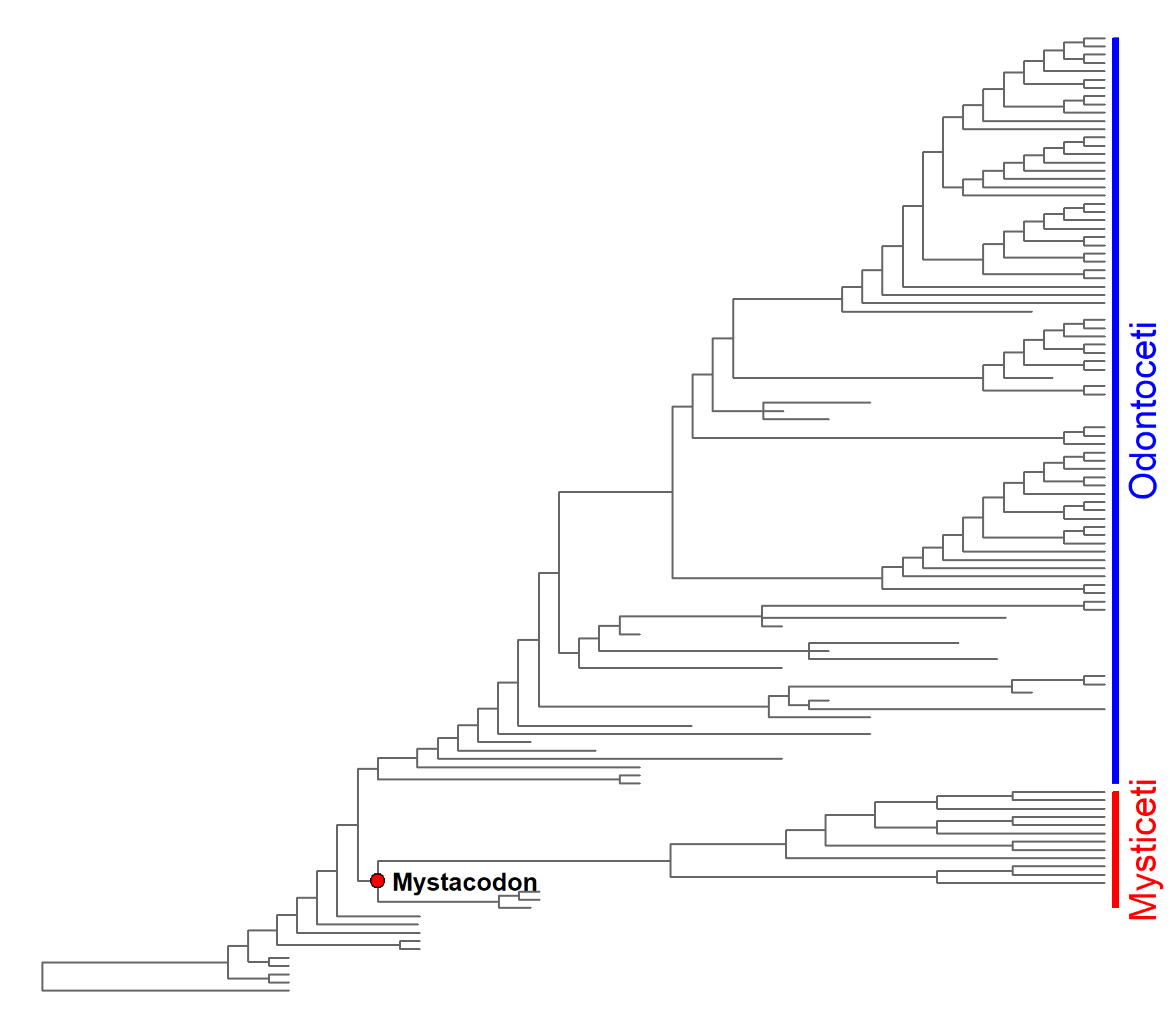# check the order of your data: best if data vectors # are sorted in the same order of the species on the phylogeny masscet[match(treecet$tip.label,names(masscet))]->masscet

## Example 1: RRphylo by accounting for the effect of a coviariate
# perform RRphylo on the vector of (log) body mass
RRphylo(tree=treecet,y=masscet)->RRmasscet

# create the covariate vector: extract phenotypic character (i.e. log body mass)
# estimates at nodes from the RR object ($aces) and collate them # to the vector of (log) body mass c(RRmasscet$aces[,1],masscet)->masscov

# perform RRphylo on the vector of (log) body mass by including
# the body mass itslef as covariate
RRphylo(tree=treecet,y=masscet,cov=masscov)->RRcov

## Example 2: RRphylo by setting values at internal nodes
# Set the body mass of Mysticetes ancestor (Mystacodon selenensis)
# as known value at node
RRphylo(tree=treecet,y=masscet,aces=aceMyst)->RRace

## Example 3: multiple regression version of RRphylo
# cross-reference the phylogenetic tree and body and brain mass data.
# Remove from both the tree and vector of body sizes the species
# whose brain size is missing
drop.tip(treecet,treecet$tip.label[-match(names(brainmasscet),treecet$tip.label)])->treecet.multi
masscet[match(treecet.multi$tip.label,names(masscet))]->masscet.multi # check the order of your data: best if # data vectors (i.e. masscet and brainmasscet) are sorted # in the same order of the species on the phylogeny masscet.multi[match(treecet.multi$tip.label,names(masscet.multi))]->masscet.multi
brainmasscet[match(treecet.multi$tip.label,names(brainmasscet))]->brainmasscet # perform RRphylo on tree and body mass data RRphylo(tree=treecet.multi,y=masscet.multi)->RRmass.multi # create the predictor vector: retrieve the ancestral character estimates # of body size at internal nodes from the RR object ($aces) and collate them
# to the vector of species' body sizes to create
c(RRmass.multi$aces[,1],masscet.multi)->x1.mass # perform the multiple regression version of RRphylo by using # the brain size as variable and the body size as predictor RRphylo(treecet.multi,y=brainmasscet,x1=x1.mass)->RRmulti ## Example 4: categorical and multiple regression version of RRphylo with ## 2 additional predictors performed by setting values at internal nodes require(phytools) set.seed(1458) # generate a random tree and a BM phenotypic vector on it rtree(50)->tree fastBM(tree)->y # produce two variables to be used as additional predictors into the multiple # regression version of RRphylo. One variable is continuous, the other is discrete. jitter(y)*10->y1 rep(1,length(y))->y2 y2[sample(1:50,20)]<-2 names(y2)<-names(y) # perform RRphylo on y1 and y2 to retrieve ancestral state estimates at nodes # and create the x1 matrix c(RRphylo(tree,y1)$aces[,1],y1)->x1
RRphylo(tree,y2)->RRcat # this is categorical RRphylo
c(RRcat\$aces[,1],y2)->x2
cbind(x1,x2)->x1mat

# create the phenotypes for y, y1, and y2 to be set as known values at internal nodes
cbind(c(jitter(mean(y1[tips(tree,83)])),1),
c(jitter(mean(y1[tips(tree,53)])),2))->acex
c(jitter(mean(y[tips(tree,83)])),jitter(mean(y[tips(tree,53)])))->acesy
names(acesy)<-rownames(acex)<-c(83,53)

# perform RRphylo by specifying aces, x1, and aces.x1 arguments
RRphylo(tree,y,aces=acesy,x1=x1mat,aces.x1 = acex)->RRcat.multi